Why you should never break a continuous algebraic loop with a Memory block

I have seen many users run into trouble when resolving an algebraic loop, so this week I want to explain why you should never break a continuous algebraic loop with a Memory block.

The Problem

Let's say I have a simple model with a control loop: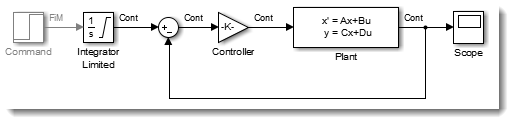If the plant model is direct feedthrough, this will result in an algebraic loop. While Simulink can solve the algebraic loop most of the time, it usually slows down the simulation, and when the solve fails to converge it can lead to errors like this: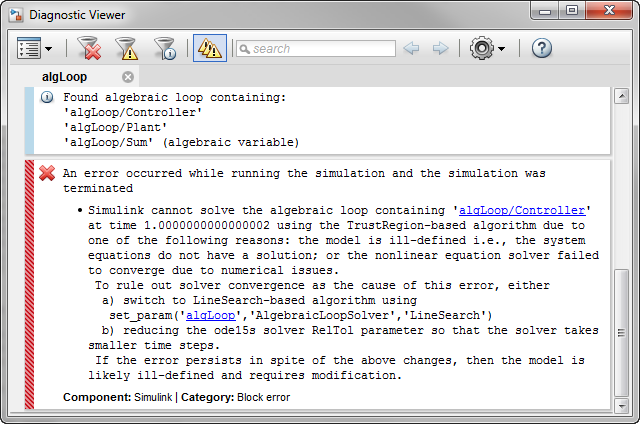Breaking the loop with a Memory Block

To break the algebraic loop, you need to insert in the loop a nondirect feedthrough block. The first thing most users think about is a Unit Delay or Memory block.

If the blocks in the algebraic loop have a discrete sample time, inserting a Unit Delay is usually the best solution. Of course this will change the dynamic of the system, this is something you need to evaluate and see if this is acceptable for your application.

If the blocks in the loop have a continuous sample time, what many users try is inserting a Memory block. The Memory block is similar to the Unit Delay block in a sense that it delays its input by one time step, however it works with variable-step signals. Let's see what it does for our model.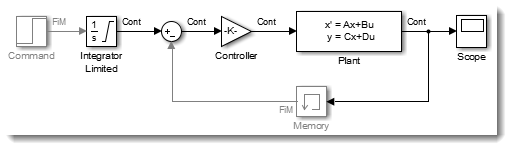At least, now the model simulates to completion and we can look at the results: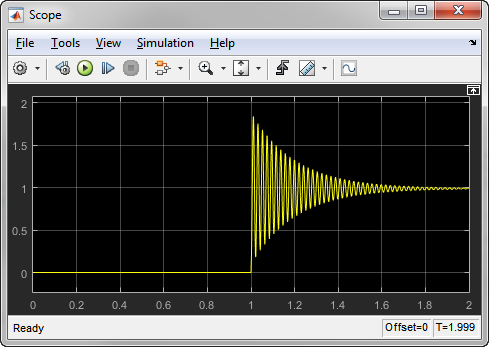However when simulating the model, we quickly notice that it simulates very slowly. If I log data from the model, I can see that it takes more than 500,000 steps to simulate this model for two seconds!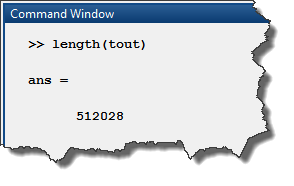If we plot the steps taken by the variable-step solver, we can see that after the step input, the solver begins to take steps of about 1e-6 seconds and gets stuck there.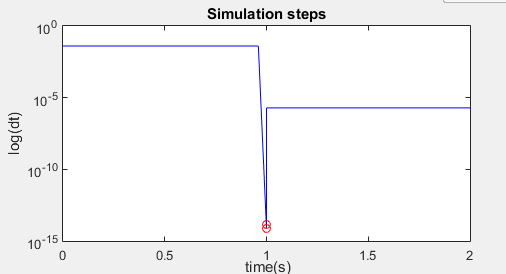Why is it happening? This is because the output of the Memory block is not continuous, and it is driving a block with continuous states, the State-Space block. Every time the output of the Memory block changes, the solver needs to reset, forcing the small step size that we observe. We know that this situation is problematic and we have a Model Advisor check for that: Check for non-continuous signals driving derivative ports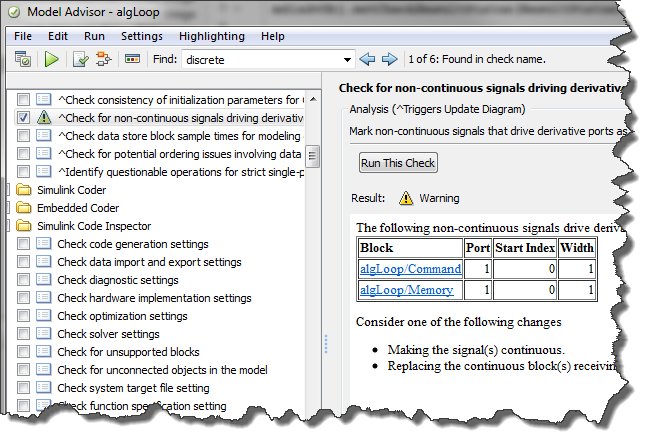The Solution: Breaking the Loop using a Transfer Function block

As suggested by the Model Advisor, the recommended way to break this algebraic loop is to use a continuous block. The one I typically prefer is a first order Transfer Function. Like the Memory block, this will introduce a new dynamic in the system. The trick is to make the time constant of the Transfer Function small enough to not affect the dynamics of the system significantly. In this case, I used 1e-6.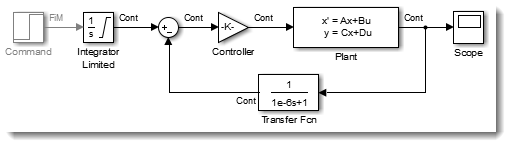With this change, the model gives similar results, but the simulation completes almost instantly, taking only 633 time steps: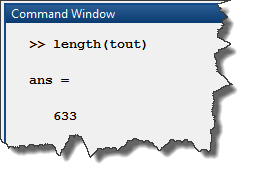If you have experiences or suggestions on how to handle algebraic loops, let us know by leaving a comment here.

*** Important Update ***

After publishing this post, a few users contacted me mentioning that some Simulink demos use a Memory block to break algebraic loops. I consequently decided to add this update to highlight the fact that breaking an algebraic loop with a Memory block is problematic only when the loop is continuous. Let's look at a few of those examples and explain why, because the loops are not continuous, it is ok to break them with a Memory block.

sldemo_clutch: In this model, the following pattern is used in the clutch logic: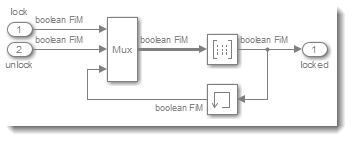You can notice that I enabled the port data type and sample time displays to highlight that this loop take a fixed-in-minor-steps sample time, and the data type of the signals involved is boolean. This subsystem implements a discrete combinatorial logic deciding if the clutch should be locked or not depending on two inputs and it's previous state. Since the loop is discrete, the Memory block is the way to go.

sldemo_bounce: In this model, we can see that an algebraic loop is broken by a Memory block:At first look, this loop has a continuous sample time and is of data type double. So why am I not considering it continuous? Because of when the loop is actually active. Let's look at the logic here. First, we need to note that the Integrator is configured to reset dx/dt when x reaches saturation: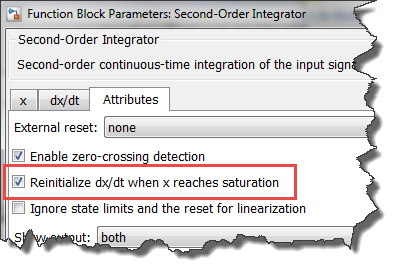As soon as the Integrator enters the saturation, we want to apply a new velocity that is 80% of the velocity when it entered the saturation, but in opposite direction, to take it out of the saturation. This means that the output of the loop is not used continuously by the Integrator. It is used only for one time step, at a discontinuity, when entering the saturation triggers a zero-crossing event.

I hope those clarification makes it clearer that when I recommend breaking algebraic loops with transfer functions, I am talking about continuous algebraic loops, where (if a Memory block was used) the output of the Memory block would drive a derivative port as can be detected by the Model Advisor check mentioned above.

|